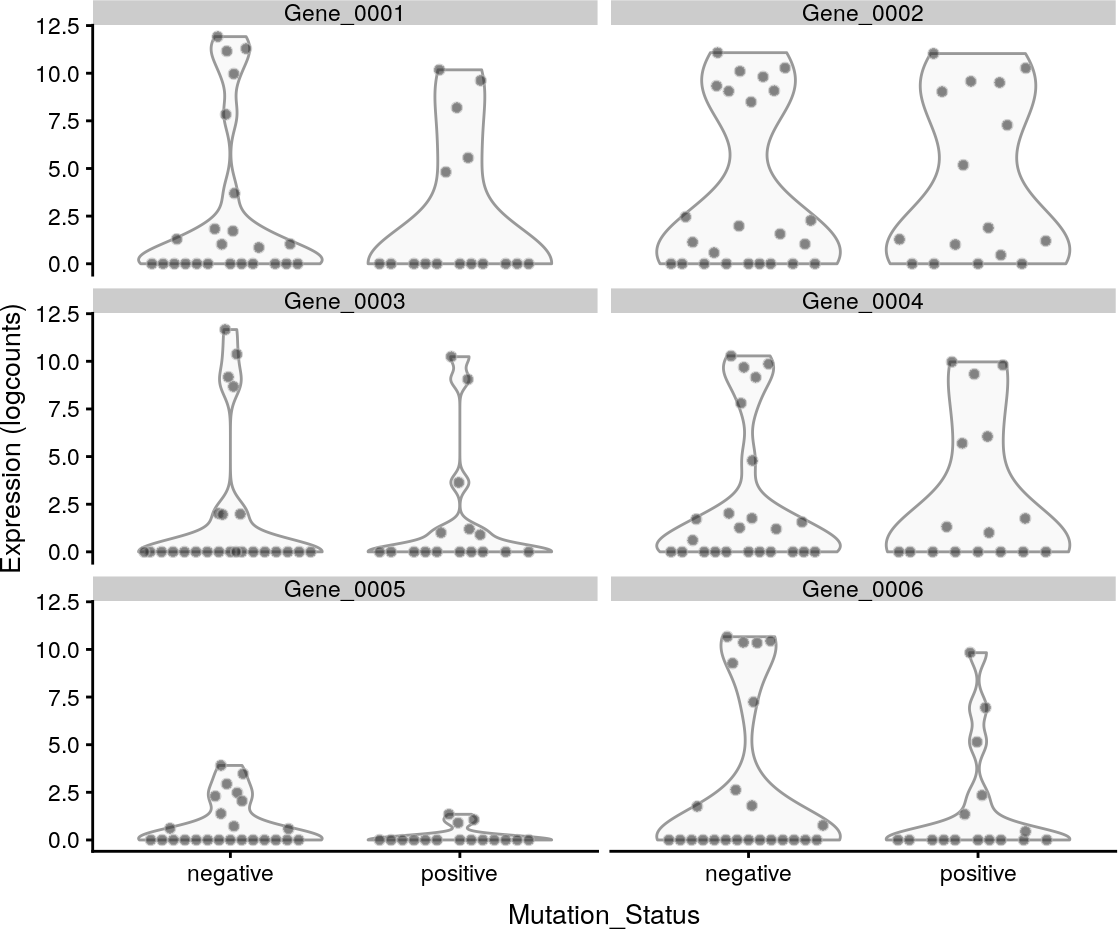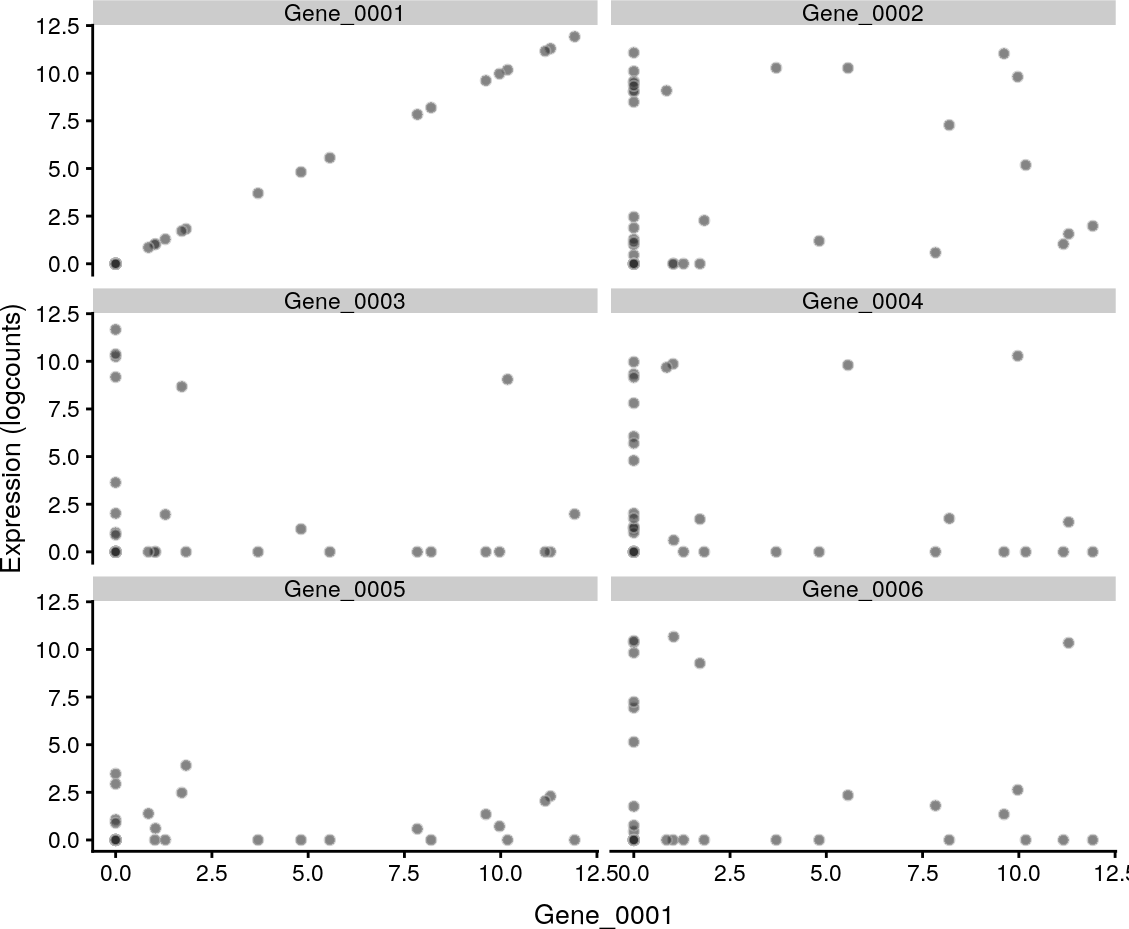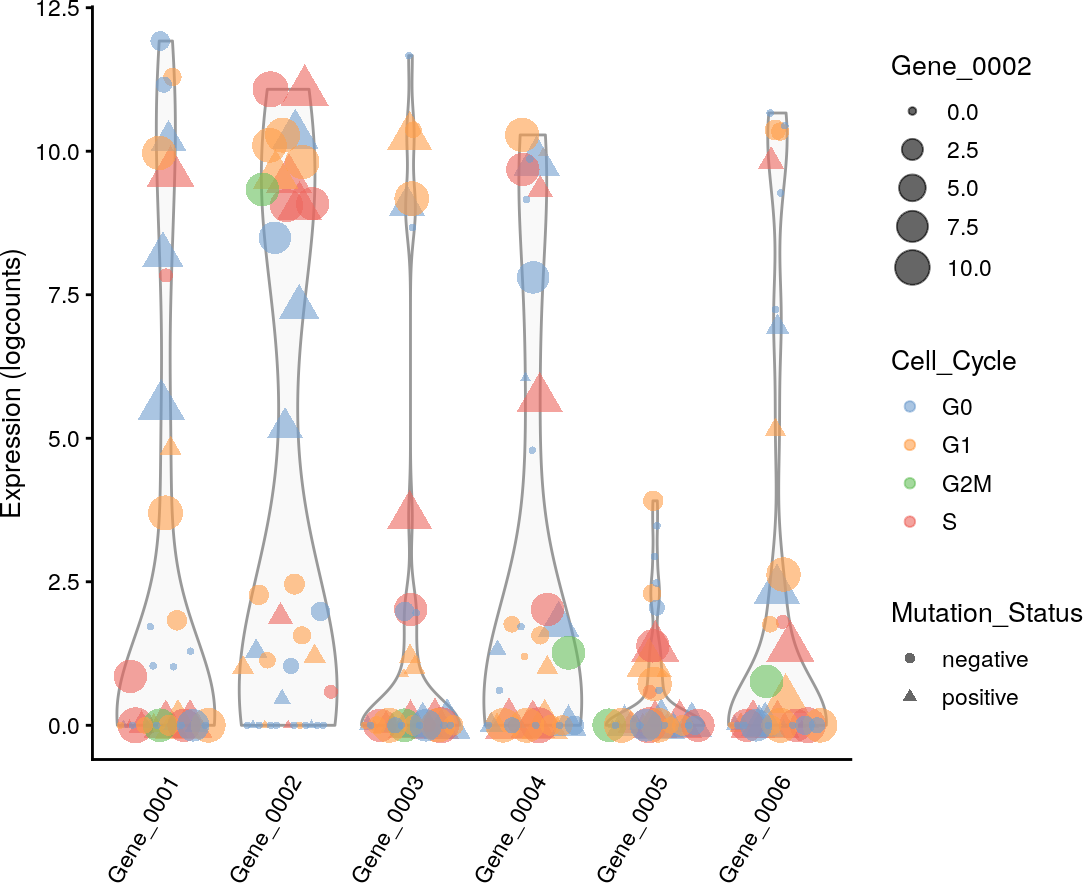# 1 Introduction

This document provides some examples of the data visualisation functions available in scater.

• `plotExpression`: plot cell expression levels for one or more genes;
• `plotReducedDim`: plot (and/or calculate) reduced dimension coordinates;
• various QC plots, described in the QC vignette.

We will demonstrate on some example data generated below:

``````library(scater)
data("sc_example_counts")
data("sc_example_cell_info")
example_sce <- SingleCellExperiment(
assays = list(counts = sc_example_counts),
colData = sc_example_cell_info
)
example_sce <- normalize(example_sce)
example_sce``````
``````## class: SingleCellExperiment
## dim: 2000 40
## assays(2): counts logcounts
## rownames(2000): Gene_0001 Gene_0002 ... Gene_1999 Gene_2000
## rowData names(0):
## colnames(40): Cell_001 Cell_002 ... Cell_039 Cell_040
## colData names(4): Cell Mutation_Status Cell_Cycle Treatment
## reducedDimNames(0):
## spikeNames(0):``````

# 2 Plots of expression values

The `plotExpression` function makes it easy to plot expression values for a subset of genes or features. This can be particularly useful for further examination of features identified from differential expression testing, pseudotime analysis or other analyses. By default, it uses expression values in the `"logcounts"` assay, but this can be changed through the `exprs_values` argument.

``````plotExpression(example_sce, rownames(example_sce)[1:6],
x = "Mutation_Status", exprs_values = "logcounts") ``````Setting `x` will determine the covariate to be shown on the x-axis. This can be a field in the column metadata or the name of a feature (to obtain the expression profile across cells). Categorical covariates will yield grouped violins as shown above, with one panel per feature. By comparison, continuous covariates will generate a scatter plot in each panel, as shown below.

``````plotExpression(example_sce, rownames(example_sce)[1:6],
x = "Gene_0001")``````The points can also be coloured, shaped or resized by the column metadata or expression values.

``````plotExpression(example_sce, rownames(example_sce)[1:6],
colour_by = "Cell_Cycle", shape_by = "Mutation_Status",
size_by = "Gene_0002")``````For categorical `x`, we can also show the median expression level per group on the plot to summarise the distribution of expression values:

``````plotExpression(example_sce, rownames(example_sce)[7:12],
x = "Mutation_Status", exprs_values = "counts",
colour = "Cell_Cycle", show_median = TRUE,
xlab = "Mutation Status", log = TRUE)``````